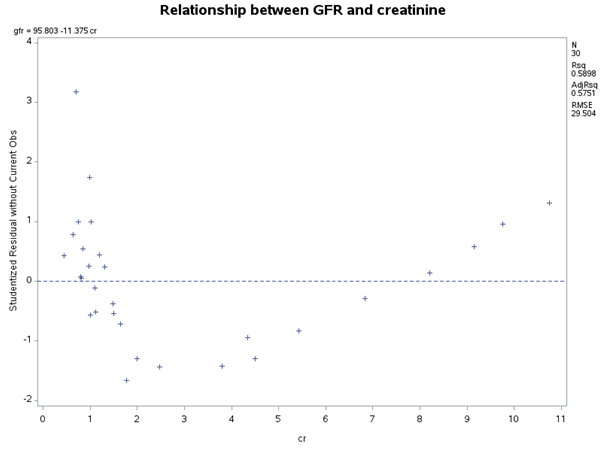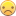## Plotting of rstudent by regressors in SAS University Edition

Hi all

I am learning SAS Studio (University edition) as we plan on using this to teach our Multiple regression course this year instead of SAS 9.3 or 4

I am trying to produce plot/s of rstudent v the regressors.

Here is an example of how I would have done this with my 'normal' SAS software.

PROC REG DATA=dataset ;

MODEL outcome = var1 var2 / R;

PLOT rstudent.*(var1 var2 predicted.);

RUN;

This would have produced three plots all with rstudent on y axis.

In SAS studio I can get the rstudent by predicted without issue as it is a standard component of the diagnostic plots (also I can code Plots = rstudentbypredicted if I wanted a bigger one).

The only plots I can get for Var1 and Var2 are for plain residuals and I can't seem to find any code for rstudent by regressor/s

Regards

Marisa

1 ACCEPTED SOLUTION

Accepted Solutions

## Re: Plotting of rstudent by regressors in SAS University Edition

Use the OUTPUT statement to save the studentized residuals and predicted values to a data set. Use PROC SGPLOT to create the graphs:

PROC REG DATA=sashelp.class plots(only)=rstudentbypredicted ;
MODEL age = height weight / R;
output out=RegOut P=Predicted RSTUDENT=RStudent;
RUN;

proc sgplot data=RegOut;
scatter x=Height y=RStudent;
run;

proc sgplot data=RegOut;
scatter x=Weight y=RStudent;
run;

10 REPLIES 10Jay54
Meteorite | Level 14

## Re: Plotting of rstudent by regressors in SAS University Edition

Can you attach a fully working program with data?  Or, a program that uses one of the data sets from SASHELP to illustrate the issue.  Also include SAS Studio release (R3.2, R3.2, etc.).  You may get better help posting to the "SAS Statistical Procedures" communities page.

## Re: Plotting of rstudent by regressors in SAS University Edition

The PLOT statement does not appear in the SAS STAT 13.2 documentation, but it does in SAS STAT 13.1. In this case I'd create an output dataset and manually plot it. Not a great solution, but a quick work around. It's probably worth knowing how to store the output in a dataset anywaysRunning on SAS University Edition I get the following error. I'd contact Tech Support directly regarding this.

39 PROC REG DATA=sashelp.class ;

40 MODEL weight = height age / R;

41 PLOT rstudent.*(height age predicted.);

42 RUN;

NOTE: The SAS System stopped processing this step because of errors.

NOTE: The PROCEDURE REG printed pages 3-4.

NOTE: PROCEDURE REG used (Total process time):

real time 4.15 seconds

cpu time 0.97 seconds

## Re: Plotting of rstudent by regressors in SAS University Edition

Hi Sanjay

Please find below the code and the plot I require that I produced from SAS 9.4.

The GFR dataset is attached.

The plots that I am having problems with are bolded.

I successfully get the standard diagnostic plots and residuals by x variables but receive the following error message from SAS Studio V 3.1

I have seen discussions regarding this error message that say you should use the PLOTS = option which I have tried but there does not appear to be an option for rstudent by x variables just rstudentbypredicted or leverage.

DATA gfr;

INFILE 'c:\data\gfr.dat';    *Use  INFILE '/folders/myfolders/gfr.dat'; for SAS studio;

INPUT id age cr gfr;

* Calculate inverse CR;

invcr = 1/cr;

RUN;

TITLE 'Relationship between GFR and creatinine';

PROC UNIVARIATE DATA = GFR PLOT;

VAR age cr gfr invcr;

RUN;

PROC REG CORR DATA = GFR ;

MODEL gfr=cr;

PLOT rstudent.*(cr predicted.);

MODEL gfr=invcr / R;

PLOT rstudent.*(invcr predicted.);

* save the residuals of the previous model in 'RESIDS';

OUTPUT OUT=resids RSTUDENT=jackknife;

RUN;

* Check the distribution of the residuals;

PROC UNIVARIATE DATA=resids NORMAL PLOT;

VAR jackknife;

QQPLOT / NORMAL (MU=est SIGMA=est);

RUN;This is the plot produced by PLOT rstudent. * cr

## Re: Plotting of rstudent by regressors in SAS University Edition

Bad designApparently it requires SAS/GRAPH which isn't licensed in SAS UE.Jay54
Meteorite | Level 14

## Re: Plotting of rstudent by regressors in SAS University Edition

Not sure of the details.  I sent it to others who know more about stat procedures.

Try adding a "ods graphics on;" statement before the call to the procedures.

## Re: Plotting of rstudent by regressors in SAS University Edition

Thanks Sanjay and Reeza

I have tried ODS graphics on and followed all instructions I could find on SAS site but still no good.

Reeza you mentioned workarounds which is fine but we are trying to teach introductory regression to students so we want it to be as standard as possible from one year to the next.

Marisa

## Re: Plotting of rstudent by regressors in SAS University Edition

I agree, not a fan of changes from version to version, but sometimes necessary.Jay54
Meteorite | Level 14

## Re: Plotting of rstudent by regressors in SAS University Edition

Here is another suggestion from stat experts.  Rick covered how to so some graphs using SGPLOT.

"She is using the old Plots statement which predates Ods graphics and produces grseg graphs. She should switch to using the Plots= option.  There may be some combinations of plots you now can't get with the option and those would need to be done with SGPlot."

Another suggestion:

proc reg data=sashelp.class;

model age = height weight / r;

output out=b p=p rstudent=rs;

run; quit;

proc sgscatter; plot rs*(height weight p); run;

## Re: Plotting of rstudent by regressors in SAS University Edition

Thank you all for your help; I have used both Rick's and Sanjay's code and have got what I need.

This plot didn't have a reference line at 0 which was a shame so added the following 'refline' code

proc sgplot;

scatter y = rstudent x = var1;

refline 0 / axis = y;

run;

Sorry for the delay in responding.

Marisa

## Re: Plotting of rstudent by regressors in SAS University Edition

Use the OUTPUT statement to save the studentized residuals and predicted values to a data set. Use PROC SGPLOT to create the graphs:

PROC REG DATA=sashelp.class plots(only)=rstudentbypredicted ;
MODEL age = height weight / R;
output out=RegOut P=Predicted RSTUDENT=RStudent;
RUN;

proc sgplot data=RegOut;
scatter x=Height y=RStudent;
run;

proc sgplot data=RegOut;
scatter x=Weight y=RStudent;
run;

Discussion stats
• 10 replies
• 4976 views
• 9 likes
• 4 in conversation